# Tag: Conway

The monstrous moonshine picture is a sub-graph of Conway’s Big Picture on 218 vertices. These vertices are the classes of lattices needed in the construction of the 171 moonshine groups. That is, moonshine gives us the shape of the picture.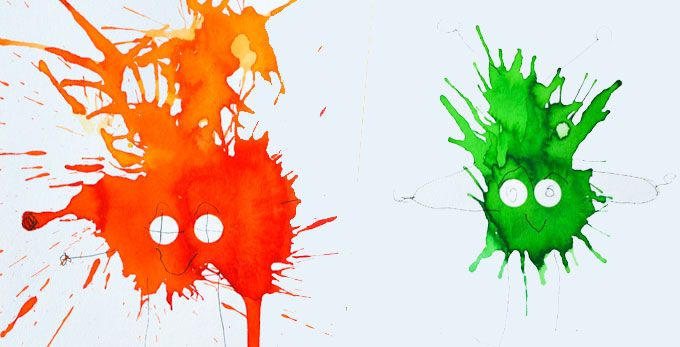(image credit Friendly Monsters)

But we can ask to reverse this process. Is the shape of the picture dictated by group-theoretic properties of the monster?

That is, can we reconstruct the 218 lattices and their edges starting from say the conjugacy classes of the monster and some simple rules?

Look at the the power maps for the monster. That is, the operation on conjugacy classes sending the class of $g$ to that of $g^k$ for all divisors $k$ of the order of $g$. Or, if you prefer, the $\lambda$-ring structure on the representation ring.

Rejoice die-hard believers in $\mathbb{F}_1$-theory, rejoice!

Here’s the game to play.

Let $g$ be a monster element of order $n$ and take $d=gcd(n,24)$.

(1) : If $d=8$ and a power map of $g$ gives class $8C$ add $(n|4)$ to your list.

(2) : Otherwise, look at the smallest power of $g$ such that the class is one of $12J,8F,6F,4D, 3C,2B$ or $1A$ and add $(n|e)$ where $e$ is the order of that class, or, if $n > 24$ and $e$ is even add $(n | \frac{e}{2})$.

A few examples:

For class 20E, $d=4$ and the power maps give classes 4D and 2B, so we add $(20|2)$.

For class 32B, $d=8$ but the power map gives 8E so we resort to rule (2). Here the power maps give 8E, 4C and 2B. So, the best class is 4C but as $32 > 24$ we add $(32|2)$.

For class 93A, $d=3$ and the power map gives 3C and even though $93 > 24$ we add $(93|3)$.

This gives us a list of instances $(n|e)$ with $n$ the order of a monster element. For $N=n \times e$ look at all divisors $h$ of $24$ such that $h^2$ divides $N$ and add to your list of lattices those of the form $M \frac{g}{h}$ with $g$ strictly smaller than $h$ and $(g,h)=1$ and $M$ a divisor of $\frac{N}{h^2}$.

This gives us a list of lattices $M \frac{g}{h}$, which is an $h$-th root of unity centered as $L=M \times h$ (see this post). If we do this for all lattices in the list we can partition the $L$’s in families according to which roots of unity are centered at $L$.

This gives us the moonshine picture. (modulo mistakes I made)

The operations we have to do after we have our list of instances $(n|e)$ is pretty straightforward from the rules we used to determine the lattices needed to describe a moonshine group.

Perhaps the oddest part in the construction are the rules (1) and (2) and the prescribed conjugacy classes used in them.

One way to look at this is that the classes $8C$ and $12J$ (or $24J$) are special. The other classes are just the power-maps of $12J$.

Another ‘rationale’ behind these classes may come from the notion of harmonics (see the original Monstrous moonshine paper page 312) of the identity element and the two classes of involutions, 2A (the Fischer involutions) and 2B (the Conway involutions).

For 1A these are : 1A,3C

For 2A these are : 2A,4B,8C

For 2B these are : 2B,4D,6F,8F,12J,24J

These are exactly the classes that we used in (1) and (2), if we add the power-classes of 8C.

Perhaps I should take some time to write all this down more formally.

The monstrous moonshine picture is the finite piece of Conway’s Big Picture needed to understand the 171 moonshine groups associated to conjugacy classes of the monster.

Last time I claimed that there were exactly 7 types of local behaviour, but I missed one. The forgotten type is centered at the number lattice $84$.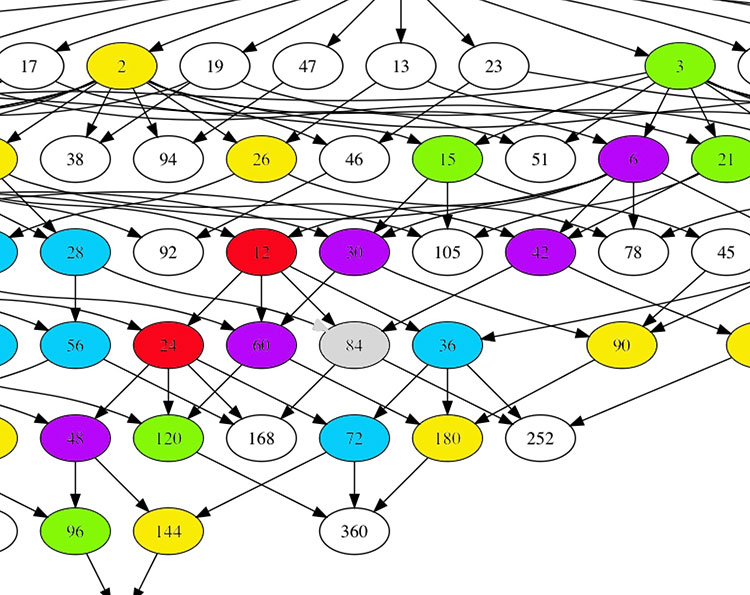Locally around it the moonshine picture looks like this
$\xymatrix{42 \ar@{-}[dr] & 28 \frac{1}{3} \ar@[red]@{-}[d] & 41 \frac{1}{2} \ar@{-}[ld] \\ 28 \ar@[red]@{-}[r] & \color{grey}{84} \ar@[red]@{-}[r] \ar@[red]@{-}[d] \ar@{-}[rd] & 28 \frac{2}{3} \\ & 252 & 168}$

and it involves all square roots of unity ($42$, $42 \frac{1}{2}$ and $168$) and $3$-rd roots of unity ($28$, $28 \frac{1}{3}$, $28 \frac{2}{3}$ and $252$) centered at $84$.

No, I’m not hallucinating, there are indeed $3$ square roots of unity and $4$ third roots of unity as they come in two families, depending on which of the two canonical forms to express a lattice is chosen.

In the ‘normal’ expression $M \frac{g}{h}$ the two square roots are $42$ and $42 \frac{1}{2}$ and the three third roots are $28, 28 \frac{1}{3}$ and $28 \frac{2}{3}$. But in the ‘other’ expression
$M \frac{g}{h} = (\frac{g’}{h},\frac{1}{h^2M})$
(with $g.g’ \equiv 1~mod~h$) the families of $2$-nd and $3$-rd roots of unity are
$\{ 42 \frac{1}{2} = (\frac{1}{2},\frac{1}{168}), 168 = (0,\frac{1}{168}) \}$
and
$\{ 28 \frac{1}{3} = (\frac{1}{3},\frac{1}{252}), 28 \frac{2}{3} = (\frac{2}{3},\frac{1}{252}), 252 = (0 , \frac{1}{252}) \}$
As in the tetrahedral snake post, it is best to view the four $3$-rd roots of unity centered at $84$ as the vertices of a tetrahedron with center of gravity at $84$. Power maps in the first family correspond to rotations along the axis through $252$ and power maps in the second family are rotations along the axis through $28$.

In the ‘normal’ expression of lattices there’s then a total of 8 different local types, but two of them consist of just one number lattice: in $8$ the local picture contains all square, $4$-th and $8$-th roots of unity centered at $8$, and in $84$ the square and $3$-rd roots.

Perhaps surprisingly, if we redo everything in the ‘other’ expression (and use the other families of roots of unity), then the moonshine picture has only 7 types of local behaviour. The forgotten type $84$ appears to split into two occurrences of other types (one with only square roots of unity, and one with only $3$-rd roots).

I wonder what all this has to do with the action of the Bost-Connes algebra on the big picture or with Plazas’ approach to moonshine via non-commutative geometry.

Do we know why the monster exists and why there’s moonshine around it?

The answer depends on whether or not you believe that vertex operator algebras are natural, elegant and inescapable objects.

the monster

Simple groups often arise from symmetries of exceptionally nice mathematical objects.

The smallest of them all, $A_5$, gives us the rotation symmetries of the icosahedron. The next one, Klein’s simple group $L_2(7)$, comes from the Klein quartic.

The smallest sporadic groups, the Mathieu groups, come from Steiner systems, and the Conway groups from the 24-dimensional Leech lattice.

What about the largest sporadic simple, the monster $\mathbb{M}$?

In his paper What is … the monster? Richard Borcherds writes (among other characterisations of $\mathbb{M}$):

“3. It is the automorphism group of the monster vertex algebra. (This is probably the best answer.)”

“Unfortunately none of these definitions is completely satisfactory. At the moment all constructions of the algebraic structures above seem artificial; they are constructed as sums of two or more apparently unrelated spaces, and it takes a lot of effort to define the algebraic structure on the sum of these spaces and to check that the monster acts on the resulting structure.
It is still an open problem to find a really simple and natural construction of the monster vertex algebra.

Here’s 2 minutes of John Conway on the “one thing” he really wants to know before he dies: why the monster group exists.

moonshine

Moonshine started off with McKay’s observation that 196884 (the first coefficient in the normalized j-function) is the sum 1+196883 of the dimensions of the two smallest simple representations of $\mathbb{M}$.

Soon it was realised that every conjugacy class of the monster has a genus zero group (or ‘moonshine group’) associated to it.

Borcherds proved the ‘monstrous moonshine conjectures’ asserting that the associated main modular function of such a group is the character series of the action of the element on the monster vertex algebra.

Here’s Borcherds’ ICM talk in Berlin on this: What is … Moonshine?.

Once again, the monster vertex algebra appears to be the final answer.

However, in characterising the 171 moonshine groups among all possible genus zero groups one has proved that they are all of the form:

(ii) : $(n|h)+e,g,\dots$

In his book Moonshine beyond the Monster, Terry Gannon writes:

“We now understand the significance, in the VOA or CFT framework, of transformations in $SL_2(\mathbb{Z})$, but (ii) emphasises that many modular transformations relevant to Moonshine are more general (called the Atkin-Lehner involutions).
Monstrous moonshine will remain mysterious until we can understand its Atkin-Lehner symmetries.

The monstrous moonshine picture is the subgraph of Conway’s big picture consisting of all lattices needed to describe the 171 moonshine groups.

It consists of:

– exactly 218 vertices (that is, lattices), out of which

– 97 are number-lattices (that is of the form $M$ with $M$ a positive integer), and

– 121 are proper number-like lattices (that is of the form $M \frac{g}{h}$ with $M$ a positive integer, $h$ a divisor of $24$ and $1 \leq g \leq h$ with $(g,h)=1$).

The $97$ number lattices are closed under taking divisors, and the corresponding Hasse diagram has the following shape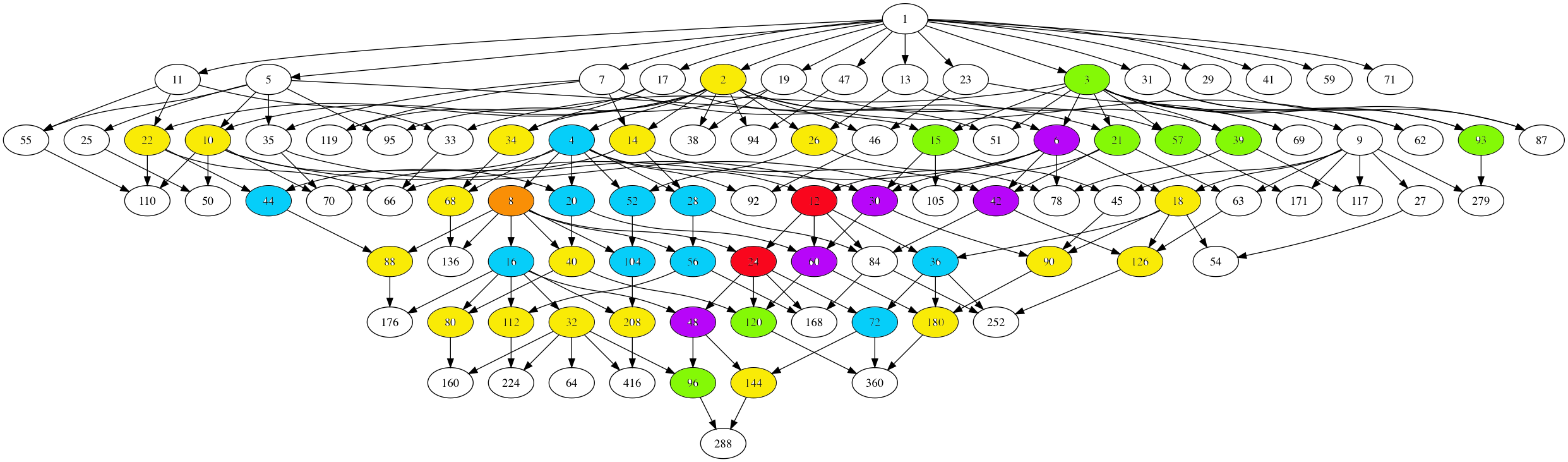Here, number-lattices have the same colour if they have the same local structure in the moonshine picture (that is, have a similar neighbourhood of proper number-like lattices).

There are 7 different types of local behaviour:

The white numbered lattices have no proper number-like neighbours in the picture.

The yellow number lattices (2,10,14,18,22,26,32,34,40,68,80,88,90,112,126,144,180,208 = 2M) have local structure

$\xymatrix{M \ar@{-}[r] & \color{yellow}{2M} \ar@{-}[r] & M \frac{1}{2}}$

which involves all $2$-nd (square) roots of unity centered at the lattice.

The green number lattices (3,15,21,39,57,93,96,120 = 3M) have local structure

$\xymatrix{& M \ar@[red]@{-}[d] & \\ M \frac{1}{3} \ar@[red]@{-}[r] & \color{green}{3M} \ar@[red]@{-}[r] & M \frac{2}{3}}$

which involve all $3$-rd roots of unity centered at the lattice.

The blue number lattices (4,16,20,28,36,44,52,56,72,104 = 4M) have as local structure

$\xymatrix{M \frac{1}{2} \ar@{-}[d] & & M \frac{1}{4} \ar@{-}[d] \\ 2M \ar@{-}[r] & \color{blue}{4M} \ar@{-}[r] & 2M \frac{1}{2} \ar@{-}[d] \\ M \ar@{-}[u] & & M \frac{3}{4}}$

and involve the $2$-nd and $4$-th root of unity centered at the lattice.

The purple number lattices (6,30,42,48,60 = 6M) have local structure

$\xymatrix{& M \frac{1}{3} \ar@[red]@{-}[d] & 2M \frac{1}{3} & M \frac{1}{6} \ar@[red]@{-}[d] & \\ M \ar@[red]@{-}[r] & 3M \ar@{-}[r] \ar@[red]@{-}[d] & \color{purple}{6M} \ar@{-}[r] \ar@[red]@{-}[u] \ar@[red]@{-}[d] & 3M \frac{1}{2} \ar@[red]@{-}[r] \ar@[red]@{-}[d] & M \frac{5}{6} \\ & M \frac{2}{3} & 2M \frac{2}{3} & M \frac{1}{2} & }$

and involve all $2$-nd, $3$-rd and $6$-th roots of unity centered at the lattice.

The unique brown number lattice 8 has local structure

$\xymatrix{& & 1 \frac{1}{4} \ar@{-}[d] & & 1 \frac{1}{8} \ar@{-}[d] & \\ & 1 \frac{1}{2} \ar@{-}[d] & 2 \frac{1}{2} \ar@{-}[r] \ar@{-}[d] & 1 \frac{3}{4} & 2 \frac{1}{4} \ar@{-}[r] & 1 \frac{5}{8} \\ 1 \ar@{-}[r] & 2 \ar@{-}[r] & 4 \ar@{-}[r] & \color{brown}{8} \ar@{-}[r] & 4 \frac{1}{2} \ar@{-}[d] \ar@{-}[u] & \\ & & & 1 \frac{7}{8} \ar@{-}[r] & 2 \frac{3}{4} \ar@{-}[r] & 1 \frac{3}{8}}$

which involves all $2$-nd, $4$-th and $8$-th roots of unity centered at $8$.

Finally, the local structure for the central red lattices $12,24 = 12M$ is

$\xymatrix{ M \frac{1}{12} \ar@[red]@{-}[dr] & M \frac{5}{12} \ar@[red]@{-}[d] & M \frac{3}{4} \ar@[red]@{-}[dl] & & M \frac{1}{6} \ar@[red]@{-}[dr] & M \frac{1}{2} \ar@[red]@{-}[d] & M \frac{5}{6} \ar@[red]@{-}[dl] \\ & 3M \frac{1}{4} \ar@{-}[dr] & 2M \frac{1}{6} \ar@[red]@{-}[d] & 4M \frac{1}{3} \ar@[red]@{-}[d] & 2M \frac{1}{3} \ar@[red]@{-}[d] & 3M \frac{1}{2} \ar@{-}[dl] & \\ & 2M \frac{1}{2} \ar@[red]@{-}[r] & 6M \frac{1}{2} \ar@{-}[dl] \ar@[red]@{-}[d] \ar@{-}[r] & \color{red}{12M} \ar@[red]@{-}[d] \ar@{-}[r] & 6M \ar@[red]@{-}[d] \ar@{-}[dr] \ar@[red]@{-}[r] & 2M & \\ & 3M \frac{3}{4} \ar@[red]@{-}[dl] \ar@[red]@{-}[d] \ar@[red]@{-}[dr] & 2M \frac{5}{6} & 4M \frac{2}{3} & 2M \frac{2}{3} & 3M \ar@[red]@{-}[dl] \ar@[red]@{-}[d] \ar@[red]@{-}[dr] & \\ M \frac{1}{4} & M \frac{7}{12} & M \frac{11}{12} & & M \frac{1}{3} & M \frac{2}{3} & M}$

It involves all $2$-nd, $3$-rd, $4$-th, $6$-th and $12$-th roots of unity with center $12M$.

No doubt this will be relevant in connecting moonshine with non-commutative geometry and issues of replicability as in Plazas’ paper Noncommutative Geometry of Groups like $\Gamma_0(N)$.

Another of my pet follow-up projects is to determine whether or not the monster group $\mathbb{M}$ dictates the shape of the moonshine picture.

That is, can one recover the 97 number lattices and their partition in 7 families starting from the set of element orders of $\mathbb{M}$, applying some set of simple rules?

One of these rules will follow from the two equivalent notations for lattices, and the two different sets of roots of unities centered at a given lattice. This will imply that if a number lattice belongs to a given family, certain divisors and multiples of it must belong to related families.

If this works out, it may be a first step towards a possibly new understanding of moonshine.

SNORT, invented by Simon NORTon is a map-coloring game, similar to COL. Only, this time, neighbours may not be coloured differently.

SNORTgo, similar to COLgo, is SNORT played with go-stones on a go-board. That is, adjacent stones must have the same colour.

SNORT is a ‘hot’ game, meaning that each player is eager to move as most moves will improve your position. In COL players are reluctant to move, because a move limits your next moves.

For this reason, SNORT positions are much harder to evaluate, and one needs the full force of Conways’s ONAG.

Here’s a SNORTgo endgame. Who has a winning strategy?, and what is the first move in that strategy?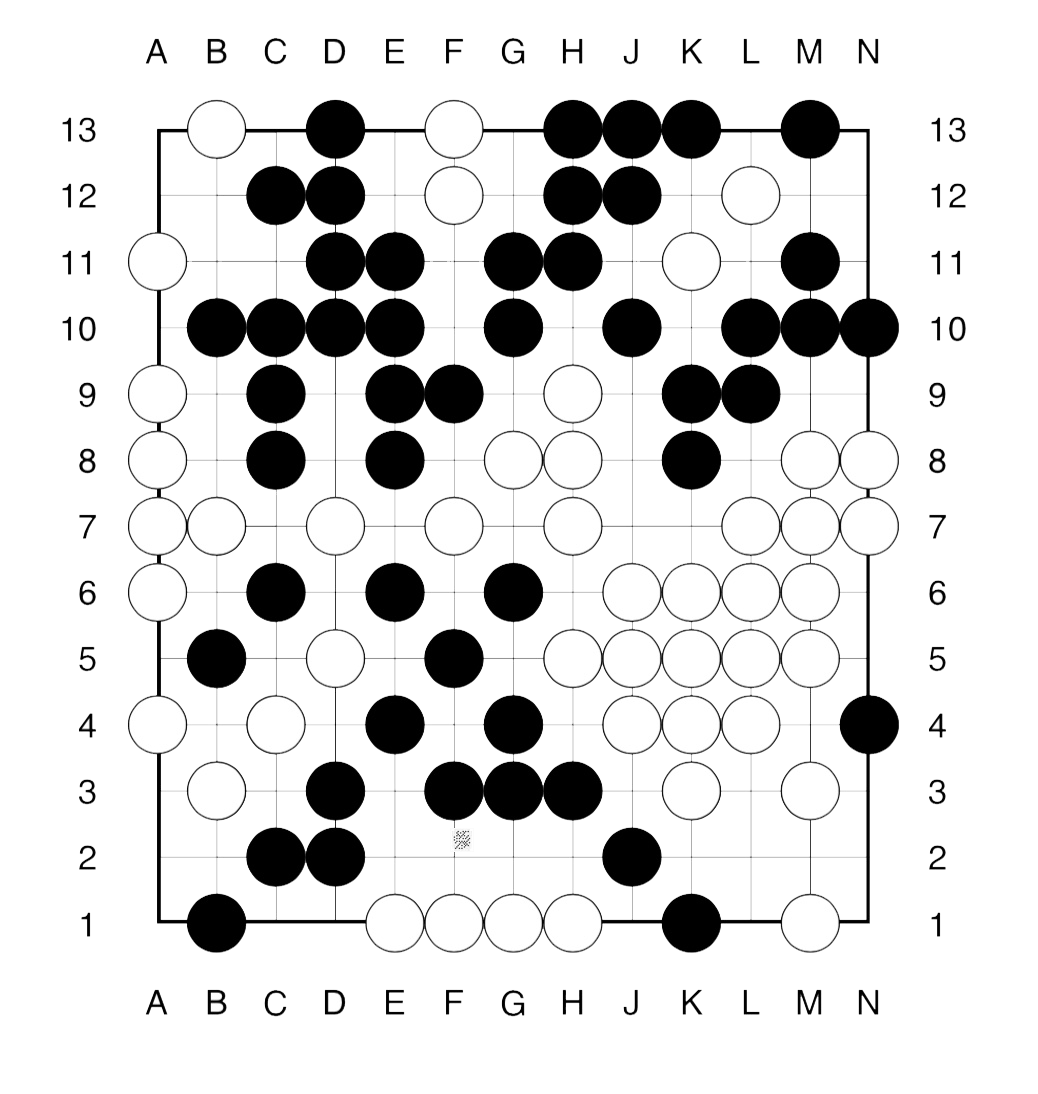The method to approach such an endgame is similar to that in COLgo. First we remove all dead spots from the board.

What remains, are a 4 spots available only to Right (white) and 5 spots available only to Left (bLack). Further, there a 3 ‘live’ regions: the upper righthand corner and the two lower corners.

The value of these corners must be computed inductively.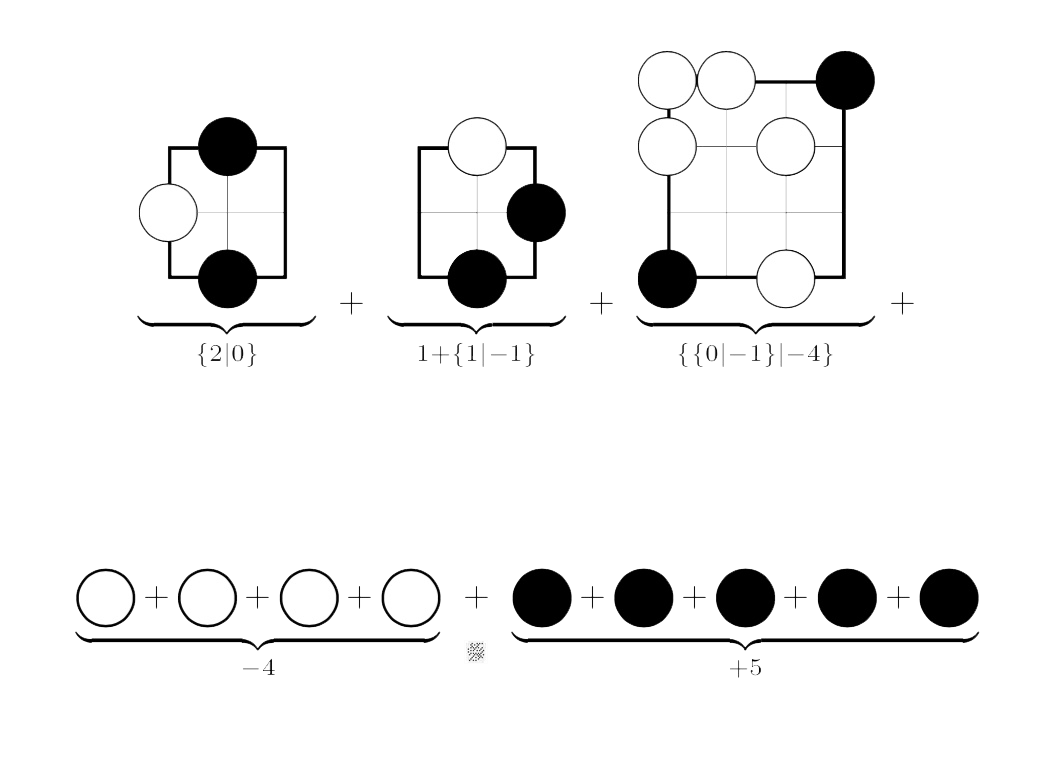For example, Right’s best option in the left-most game (corresponding to the upper righthand corner of the endgame) is to put his stone on N12, resulting in a game in which neither player can move (the zero game).

On the other hand, Left can put a stone at either N11, N12 or N13 leaving a game in which she has two more moves, whereas Right han none (the $2$ game).

The other positions are computed similarly.

To get the value of the endgame we have to sum up all these values.

This can either be done using the addition rule given in ONAG, or by using programs in combinatorial game theory.

There’s Combinatorial Game Suite, developed by Aaron Siegel. But, for some reason I can no longer use it on macOS High Sierra.

Fortunately, the older program David Wolfe’s toolkit is still available, and runs on my MacBook.

The sum game evaluates to $\{ \{3|2 \}|-1 \}$, which is a ‘fuzzy’ game, that is, its value is confused with $0$.

This means that the first player to move has a winning strategy in the endgame.

Can you spot the (unique) winning move for Right (white) and one (of two) winning move for Left (bLack)?